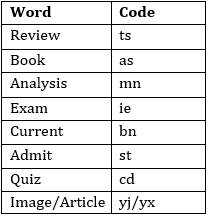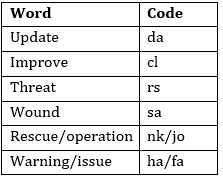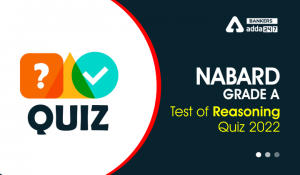Latest Banking jobs   »   Reasoning Ability Quiz For SBI Clerk...

# Reasoning Ability Quiz For SBI Clerk Prelims 2021- 27th May

Directions (1-5): Study the following information carefully and answer the given questions.
In a certain code language,
‘review exam analysis book’ is coded as ‘ts ie mn as’
‘current admit exam analysis’ is coded as ‘mn bn st ie’
‘current quiz analysis book’ is coded as ‘cd as mn bn’
‘review book image article’ is coded as ‘ts as yj yx’

Q1. Which of the following is the code for ‘admit’?
(a) st
(b) bn
(c) ie
(d) mn
(e) None of these

Q2. Which of the following quiz is coded as ‘bn’?
(a) quiz
(b) book
(d) Current
(e) None of these

Q3. What may be the code of ‘quiz orange’?
(a) st ie
(b) cd mn
(c) ie bn
(d) mn ie
(e) cd qw

Q4. Which of the following code is coded for ‘Exam’?
(a) mn
(b) ie
(c) bn
(d) cd
(e) None of these

Q5. What will be the code of ‘article?
(a) yj
(b) as
(c) yx
(d) Either (a) or (c)
(e) None of these

Directions (6-10): Study the following information carefully and answer the questions given below:
In a certain code language:
‘rescue wound operation improve’ is written as ‘cl sa nk jo’,
‘update warning issue threat’ is written as ‘ha fa rs da’,
‘improve threat wound update’ is written as ‘sa rs cl da’ and
‘rescue improve operation update’ is written as ‘cl nk jo da’.

Q6. What is the code for ‘improve threat’ in the given code language?
(a) cl fa
(b) cl rs
(c) da fa
(d) rs da
(e) None of these

Q7. What is the code for ‘warning’ in the given code language?
(a) ha
(b) fa
(c) rs
(d) da
(e) Can’t be determined

Q8. What is the code for ‘Update’ in the given code language?
(a) ha
(b) fa
(c) rs
(d) da
(e) None of these

Q9. What may be the code for ‘rescue person warning’ in the given coding language?
(a) jo nk cd
(b) nk fa rs
(c) nk ct fa
(d) ha cl sa
(e) None of these

Q10. What is the code for ‘operation’ in the given coding language?
(a) jo
(b) cl
(c) nk
(d) Either (a) or (c)
(e) ha

Directions (11-15): Study the following information carefully and answer the questions given below.
In a certain code language
‘expose explain terror’ is written as ‘ru ri be’,
‘region power updated’ is written as ‘ta no pa’,
‘climb economy terror power’ is written as ‘do ri pa pi’, and
‘region economy expose explain’ is written as ‘ru be do no’.

Q11. What is the code for ‘explain’?
(a) ri
(b) be
(c) ru
(d) do
(e) Can’t be determined

Q12. What does ‘ru’ stand for?
(a) expose
(b) Either ‘explain’ or ‘expose’
(c) terror
(d) region
(e) None of these

Q13. Which of the following is the code for ‘region power updated expose terror’?
(a) no pa ri ta do
(b) ta ri pa no be
(c) ta no ru ri pa
(d) Either (b) or (c)
(e) None of these

Q14. ‘pi ri no’ is the code for which of the following?
(a) climb region terror
(b) climb economy region
(c) explain terror economy
(d) climb terror updated
(e) None of these

Q15. Which of the following code stands for ‘power’?
(a) ri
(b) no
(c) pa
(d) do
(e) None of these

Practice More Questions of Reasoning for Competitive Exams:

###### SBI Clerk Study Plan 2021

Solutions

Solution (1-5):
Sol.S1. Ans.(a)
S2. Ans.(d)
S3. Ans.(e)
S4. Ans.(b)
S5. Ans.(d)

Solution (6-10):
Sol.S6. Ans.(b)
S7. Ans.(e)
S8. Ans.(d)
S9. Ans.(c)
S10.Ans.(d)

Solutions (11-15):
Sol.S11. Ans.(e)
S12. Ans.(b)
S13. Ans.(d)
S14. Ans.(a)
S15. Ans.(c)#### Congratulations!Incorrect details? Fill the form again here

•Reasoning Ability Quiz For IBPS RRB PO C...
•Reasoning Ability Quiz For IBPS Clerk Pr...
•Reasoning Ability Quiz For NABARD Grade ...
•Reasoning Ability Quiz For IBPS Clerk Pr...
•Reasoning Ability Quiz For IBPS Clerk Pr...
•Reasoning Ability Quiz For NABARD Grade ...